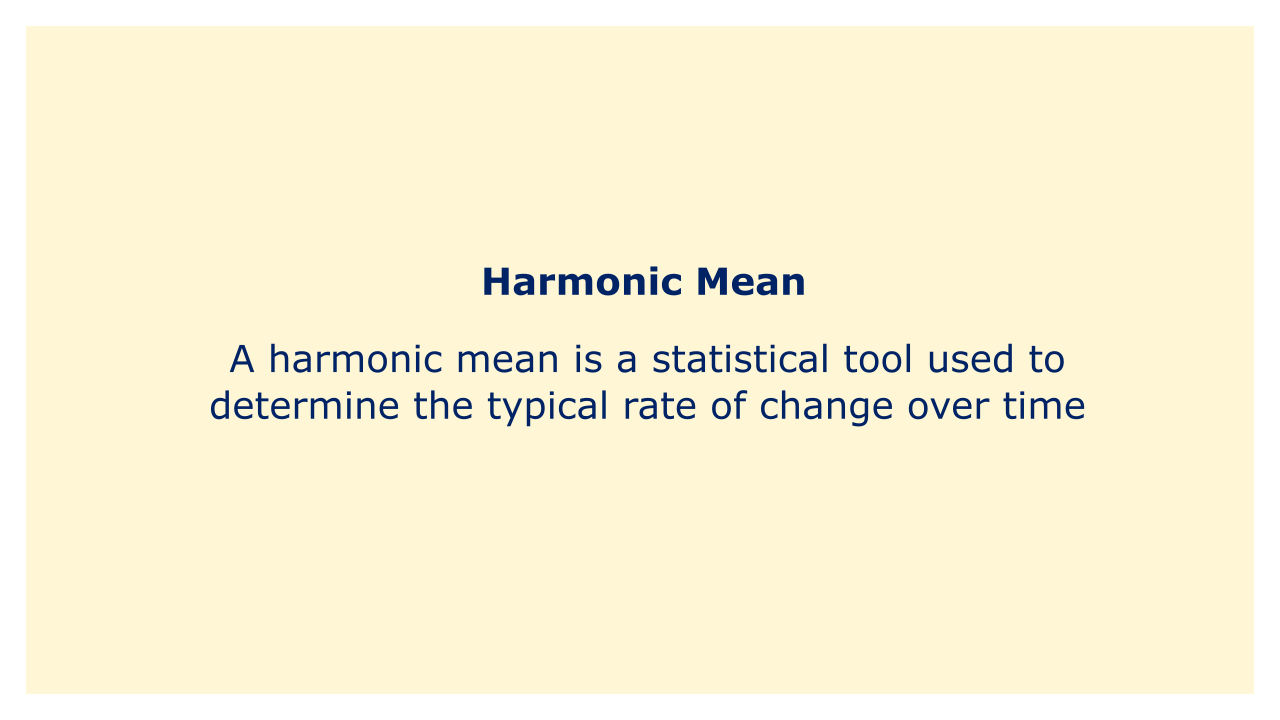# Harmonic MeanImage: Moneybestpal.com

### In finance and economics, a harmonic mean is a statistical tool used to determine the typical rate of change over time. It is the reciprocal of a set of numbers' reciprocals' arithmetic mean.

The harmonic mean is frequently used in financial analysis to determine the average return on investment over a period of time, such as the yearly return on a mutual fund over a number of years. This is due to the harmonic mean's tendency to give greater weight to periods with lower returns, which can be advantageous in some investment types where there may be years with high returns and years with poor returns.

Think of a mutual fund, for instance, where your investment yields 5% in the first year, 10% in the second year, and 15% in the third. The harmonic mean return for this investment would be:

1 / [(1/1.05) + (1/1.10) + (1/1.15)] = 10.21%

This indicates that your average annual return would have been 10.21% if you had invested in this mutual fund for the whole three years.

The harmonic mean can be helpful in some situations, but it's crucial to remember that it's not always the best technique to gauge central tendency. It may not adequately reflect the main trend of the data and is quite sensitive to outliers. As a result, while examining data, it is crucial to take additional statistical metrics such as the arithmetic mean and median into account.
Tags